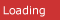# C# CornerRelated resources for scikit learn
• Multiple Linear Regression11/11/2019 3:43:29 AM. This is the fifteenth article in the series. Multiple linear regression (MLR), also known simply as multiple regression, is a statistical technique that uses several explanatory variables to predict t
• Logistic Regression11/10/2019 8:51:05 AM. This is the fourteenth tutorial in the series. Logistic regression is a statistical model that in its basic form uses a logistic function to model a binary dependent variable, although many more compl
• Linear Regression10/30/2019 2:32:26 AM. This is the thirteenth tutorial in the series. In statistics, linear regression is a linear approach to modeling the relationship between a scalar response and one or more explanatory variables. The c
• A Complete Python Scikit Learn Tutorial9/24/2019 1:40:13 AM. This is the fifth tutorial in the series. In this tutorial, we will be studying about scikit-learn and its functionalities.
• Linear Regression Using Python (SciKit Learn)11/6/2017 12:18:37 AM. Perform Linear Regression using Machine Learning Package Sci-Kit Learn (SK Learn)